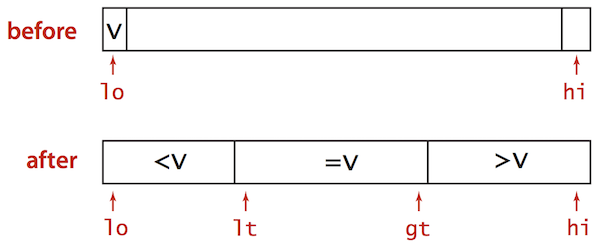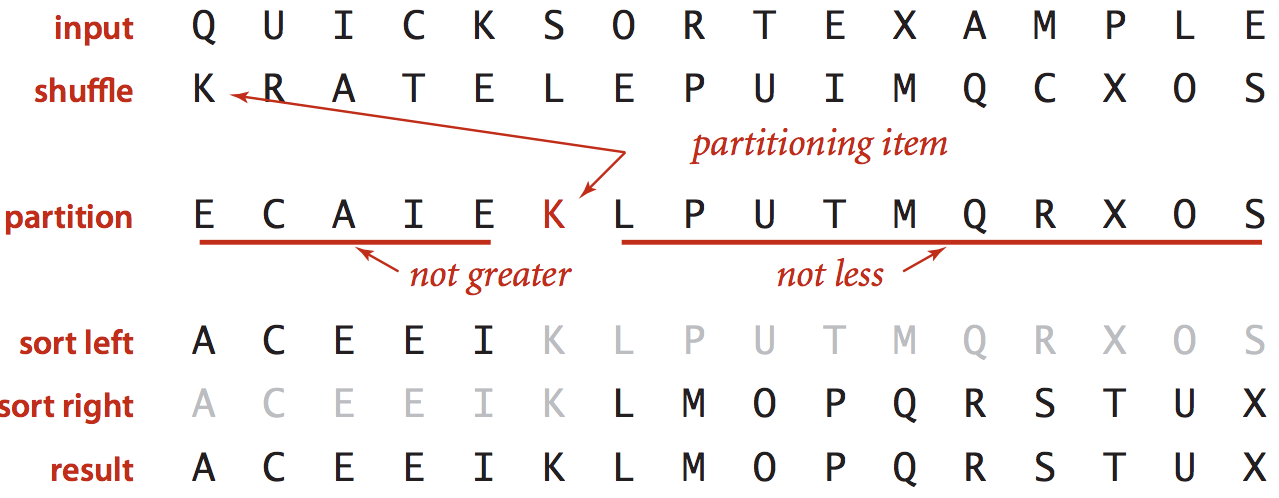Nothing special here. It’s just a blog post for summarising my algorithm learning course. Although this was already taught in the University, I remember nothing about it because I haven’t touched it for the long time.

# Quick Sort - Duplicate Keys Problem

• Quick Sort goes quadratic unless partitioning stops on equal keys!
• ~½N2 compares when all keys equal.
• B A A B A B B B C C C
• A A A A A A A A A A A
• Solve by using 3-way partitioning

# 3-way Partitioning

Partition array into 3 parts so that:

• Entries between lt and gt equal to partition item v
• No larger entries to left of lt
• No smaller entries to right of gt• Let v be partitioning item a[lo]
• Scan i from left to right.
• (a[i] < v): exchange a[lt] with a[i]; increment both lt and i
• (a[i] > v): exchange a[gt] with a[i]; decrement gt
• (a[i] == v): increment i

Nothing special here. It’s just a blog post for summarising my algorithm learning course. Although this was already taught in the University, I didn’t even know that it can be used for Selection Problem

# Selection Problem

Given an array of N items, find a kth smallest item. For example, an array A = [5, 2, 9, 4, 10, 7], the 3rd smallest item is 5, the 2nd smallest item is 4 and the smallest item is 2

# The idea

• Based on Quick Sort
• Partition the array so that
• Entry a[j] is in place.
• No larger entry to the left of j
• No smaller entry to the right of j
• Repeat in one sub-array, depending on j, finished when j equals k

Nothing special here. It’s just a blog post for summarising my algorithm learning course. Although this was already taught in the University, I remember nothing about it because I haven’t touched it for the long time.

# Quick Sort

The Idea

• Shuffle the array.
• Select one item, can be the first item or last item as the pivot (the partitioned item).
• Partition the array into 2 parts, so that
• The pivot entry is in the right place
• No larger entry to the left of the pivot item
• No smaller entry to the right of pivot item
• Sort each piece recursively.Nothing special here. It’s just a blog post for summarising my algorithm learning course. Here are some questions related to merge sort.

# Merging with smaller auxiliary array

Question

Suppose that the subarray a to a[n-1] is sorted and the subarray a[n] to a[2*n-1] is sorted. How can you merge the two subarrays so that a to a[2*n-1] is sorted using an auxiliary array of length n (instead of 2n)?

Instead of copying the whole array into the auxiliary array, copy only the left half to that auxiliary array (a to a[n-1]). At this time, the first half of the original array is free to overwrite any value. Apply merge sort and write the result back to the original array, from the first position. You will always have enough space in the original array to do that.

# Counting inversions

Question

An inversion in an array a[] is a pair of entries a[i] and a[j] such that i<j but a[i]>a[j]. Given an array, design a linearithmic algorithm to count the number of inversions.

Nothing special here. It’s just a blog post for summarising my algorithm learning course. Although this was already taught in the University, it’s still good to summarise here

# Merge Sort

• Divide array into two halves.
• Sort each half.
• For each half, continue dividing into 2 halves and do merge sort.
• Merge two halves.

Here is the sample implementation from the Coursera course. Actually, I’m more familiar with the while version.

public class Merge {
private static void merge(Comparable[] a, Comparable[] aux, int lo, int mid, int hi) {
for (int k = lo; k <= hi; k++)
aux[k] = a[k];

int i = lo, j = mid + 1;
for (int k = lo; k <= hi; k++) {
if (i > mid) a[k] = aux[j++];
else if (j > hi) a[k] = aux[i++];
else if (less(aux[j], aux[i])) a[k] = aux[j++];
else a[k] = aux[i++];
}
}

private static void sort(Comparable[] a, Comparable[] aux, int lo, int hi) {
if (hi <= lo) return;
int mid = lo + (hi - lo) / 2;
sort(a, aux, lo, mid);
sort(a, aux, mid + 1, hi);
merge(a, aux, lo, mid, hi);
}

public static void sort(Comparable[] a) {
aux = new Comparable[a.length];
sort(a, aux, 0, a.length - 1);
}
}


Nothing special here. It’s just a blog post for summarising my algorithm learning course. Although some of them were already taught in the University, it’s still good to summarise here

# 1. Selection Sort

• In iteration i, find index min of smallest remaining entry.
• Swap a[i] and a[min]class Selection {
public static void sort(Comparable[] a) {
int N = a.length;
for (int i = 0; i < N; i++) {
int min = i;
for (int j = i + 1; j < N; j++)
if (less(a[j], a[min]))
min = j;
swap(a, i, min); // swap the 2 items
}
}
}


Nothing special here. It’s just a blog post for summarising my algorithm learning course. Although this was already taught in the University, it’s still good to summarise here

# 1. StacksLast In First Out

Maintain a pointer to the first item of the linked-list. Add or remove are simply to update that pointer.

public class LinkedStackOfStrings {
private Node first = null;

private class Node {
String item;
Node next;
}

public void push(String item) {
Node oldfirst = first;
first = new Node();
first.item = item;
first.next = oldfirst;
}

public String pop() {
String item = first.item;
first = first.next;
return item;
}
}


So, my friend gave me this exercise and asked me to solve it. Sometimes it’s fun to solve algorithm problem… sometimes…

Given an array A of n integer numbers, find all the (i,j) pairs such that A[i] + A[i+1] + A[i+2] + … + A[j] = 6. For example, A = [1, 5, 4, -2, 4, 8, 7, 9, 0], these pairs are valid i,j (0,1) and (2,4) because sum(A[0->1])=6 and sum(A[2->4])=6

Of course, the worst solution is to perform n2 operations. For each number in A, do another loop inside that to find the sub-sequence having the sum = 6.

Surely there should be a better solution. And here is the O(n) solution.

Nothing special here. It’s just a blog post for summarising my algorithm learning course.

# Question

Suppose that you have an nnn-story building (with floors 1 through nnn) and plenty of eggs. An egg breaks if it is dropped from floor T or higher and does not break otherwise. Your goal is to devise a strategy to determine the value of T given the following limitations on the number of eggs and tosses

# Solution 1

1 egg, ≤T tosses

This is an O(n) solution. Just do a simple loop from the first floor until the egg break.

# Solution 2

∼1lgN eggs, ∼1lgN tosses

Use Binary search strategy.

• Start from the middle floor, drop the egg
• If it breaks, repeat with the lower half
• Otherwise, repeat with the upper half

# Solution 3

∼lgT eggs, ∼2lgT tosses

An interviewer once asked me this question. At first, I was a bit nervous and thought that this is a very tricky question. It took me a while to figure out the solution and turned out this can be converted to a simpler algorithm we already learned in University.

# Question

Given a computer with only 1MB of RAM and unlimited hard disk space, sort a file which contain 1 million 8-bit integers, each on one line.

# Solution

The problem with this is our computer is limited in memory. We cannot read all 1 million integers at once into the memory. That means we can only read some small parts of the file, which leads me to a divide and conquer solution like this. The basic idea is to divide the large problem into smaller one, process each of them and then try to merge the result.

• First, read a number of integers that can fit into the memory. Actually, you should read the number of integers that can fit in the RAM/2 space (which is 512KB in this case). The reason is in the next part
• Perform any sort algorithm that you can think of on that array. Because you need another array for storing the result, you can only read only the number of integers that can fit in half of your RAM.
• In this case, each 8-bit integer is 2 bytes, so each time you can only retrieve maximum 512KB * 1024 / 2B = 262,144 integers.
• After the array is sorted, write them back to a file.
• Repeat the above steps until you finally process all the integers in the source file.
• You will need to read 4 times in this case
• Now you have a collection of sorted integers files. The next step is to apply merge sort on those file to produce the final output file. The merge sort algorithm is like this
• Read the 2 sorted files, line by line
• Compare the 2 values
• Compare which one is smaller, write to the output file
• Repeat the above steps until we reach the end one 1 of the 2 files
• Write back all the remaining values of the other files to the output file

• 2 of 17